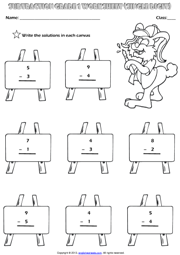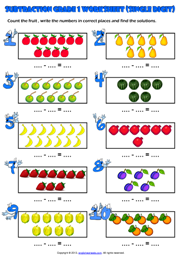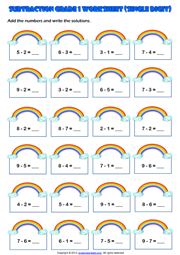Home Maths Main Page

# Grade 1 Subtraction Printable Maths Worksheets and Exercises

## Single Digit Subtraction Maths Exercise Worksheet

A fun single digit subtraction exercise maths worksheet for grade 1 (first grade) students and kids with rabbit and canvas theme. Write the solutions and colour the rabbit. (Vertical Subtraction Worksheet for kids)### Count and Subtract Picture Maths Exercise Worksheet

Count the fruits, fill in the blanks with their numbers and find the solutions. A colorful subtraction exercise maths worksheet with fruit theme for grade 1 (first grade) students and kids. (Horizontal Subtraction Worksheet for first grade students with enjoyable pictures)#### First Grade Single Digit Subtraction Math Exercise Worksheet

A simple single digit subtraction exercise maths worksheet for grade 1 (first grade) students and kids with rainbow theme. Subtract the numbers and write the solutions. (Horizontal Subtraction Worksheet for first grade students)##### First Grade Single Digit Subtraction Maths Exercise Handout
A practical subtraction exercise maths handout for grade 1 (first grade) students and kids with dogs theme. Subtract the numbers and write the solutions. (Vertical Subtraction Worksheet for first grade students)Next Topic Maths Main Page Home